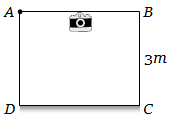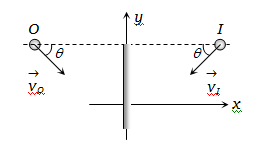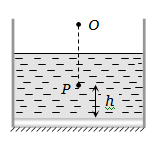A telescope has an objective lens of 10 cm diameter and is situated at a distance of one kilometre from two objects. The minimum distance between these two objects, which can be resolved by the telescope, when the mean wavelength of light is 5000 Å, is of the order of

(a) 0.5 m                        (b) 5 m

(c) 5 mm                        (d) 5 cm

Concept Questions :-

Resolution of Optical devices
High Yielding Test Series + Question Bank - NEET 2020

Difficulty Level:

Two point white dots are 1mm apart on a black paper. They are viewed by eye of pupil diameter 3 mm. Approximately, what is the maximum distance at which dots can be resolved by the eye ? [Take wavelength of light = 500 nm]

(a) 6 m                           (b) 3 m

(c) 5 m                            (d) 1 m

Concept Questions :-

Human eye
High Yielding Test Series + Question Bank - NEET 2020

Difficulty Level:

A room (cubical) is made of mirrors. An insect is moving along the diagonal on the floor such that the velocity of image of insect on two adjacent wall mirrors is . The velocity of image of insect in ceiling mirror is

(a) 10 $cm{s}^{-1}$                            (b) 20 $cm{s}^{-1}$

(c) $\frac{10}{\sqrt{2}}cm{s}^{-1}$                          (d) $10\sqrt{2}cm{s}^{-1}$

Concept Questions :-

Reflection at plane surface
High Yielding Test Series + Question Bank - NEET 2020

Difficulty Level:

Figure shows a cubical room ABCD with the wall CD as a plane mirror. Each side of the room is 3m. We place a camera at the midpoint of the wall AB. At what distance should the camera be focussed to photograph an object placed at A(a) 1.5 m                                     (b) 3 m

(c) 6 m                                         (d) More than 6 m

Concept Questions :-

Reflection at plane surface
High Yielding Test Series + Question Bank - NEET 2020

Difficulty Level:

If an object moves towards a plane mirror with a speed v at an angle $\theta$ to the perpendicular to the plane of the mirror, find the relative velocity between the object and the image(a) v                                            (b) 2v

(c) 2v cos $\theta$                                    (d) 2v sin $\theta$

Concept Questions :-

Reflection at plane surface
High Yielding Test Series + Question Bank - NEET 2020

Difficulty Level:

A plane mirror is placed at the bottom of the tank containing a liquid of refractive index $\mu$. P is a small object at a height h above the mirror. An observer vertically above P outside the liquid sees P and its image in the mirror. The apparent distance between these two will be-1. $2\mu h$

2.  $\frac{2h}{\mu }$

3. $\frac{2h}{\mu -1}$

4.  $h\left(1+\frac{1}{\mu }\right)$

Concept Questions :-

Refraction at plane surface
High Yielding Test Series + Question Bank - NEET 2020

Difficulty Level:

A thin prism having refracting angle ${10}^{°}$ is made of glass of refractive index 1.42. This prism is combined with another thin prism of glass of refractive index 1.7. This combination produces dispersion without deviation. The refracting angle of second prism should be

(a)${4}^{°}$

(b)${6}^{°}$

(c)${8}^{°}$

(d)${10}^{°}$

Concept Questions :-

Prisms
High Yielding Test Series + Question Bank - NEET 2020

Difficulty Level:

A beam of light from a source L is incident normally on a plane mirror fixed at a certain distance x from the source. The beam is reflected back as a spot on a scale placed just above the source L. When the mirror is rotated through a small angle $\theta$, the spot of light is found to move through a distance y on the scale. The angle $\theta$ is given by

(a) $\frac{y}{2x}$

(b) $\frac{y}{x}$

(c) $\frac{x}{2y}$

(d) $\frac{x}{y}$

Concept Questions :-

Reflection at plane surface
High Yielding Test Series + Question Bank - NEET 2020

Difficulty Level:

The ratio of resolving powers of an optical microscope for two wavelengths  is

(a) 8:27

(b) 9:4

(c) 3:2

(d) 16:81

Concept Questions :-

Resolution of Optical devices
High Yielding Test Series + Question Bank - NEET 2020

Difficulty Level:

Two identical glass $\left({\mu }_{g}=3/2\right)$ equi-convex lenses of focal length $f$ each are kept in contact. The space between the two lenses is filled with water $\left({\mu }_{w}=4/3\right)$. The focal length of the combination is

(a)  $f/3$             (b) $f$

(c) $\frac{4f}{3}$               (d) $\frac{3f}{4}$

Concept Questions :-

Lenses